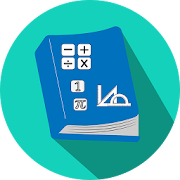# All Math Formula - Lumos Educational App Store4.21
Price -FREE
\$0

#### DESCRIPTION:

All essential Math Formula for all android users. Offline content. You don't need internet to read formulas. In this app, you will get 1000+ math formulas and equations. With formula, you will get proper diagram, so that you will understand formula easily. You can read various equations easily from this app. In this app, you will get mathematics formula and equations includes: Algebra Geometry Trigonometry Calculus: Limits Derivatives Integrals Basic Properties & Facts Arithmetic Operations Exponent Properties Properties of Radicals Properties of Inequalities Prop

#### OVERVIEW:

All Math Formula is a free educational mobile app By .It helps students in grades HS practice the following standards HSN.Q.A.2.

This page not only allows students and teachers download All Math Formula but also find engaging Sample Questions, Videos, Pins, Worksheets, Books related to the following topics.

1. HSN.Q.A.2 : Define appropriate quantities for the purpose of descriptive modeling.

HS

#### STANDARDS:

HSN.Q.A.2

Developer:

Software Version: 1.8

Category: Education

### RELATED APPSEdSearch WebSearch